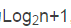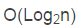• 定义好数组长度和待排数组，其中A用作暂...最好时间复杂度： O(n) 最坏时间复杂度: O(n^2) 空间复杂度： O(1) void InsertSort() { int i, j; for (int i = 2; i < N; i++) { //从第二个元素开始 if (A[i]
定义好数组长度和待排数组，其中A用作暂存空间，或者哨兵
const int N = 9;
int A[N] = { 0,49,38,65,97,76,13,27,49 };

插入排序
稳定性：        稳定
最好时间复杂度： O(n)
最坏时间复杂度:  O(n^2)
空间复杂度：     O(1)

void InsertSort() {
int i, j;
for (int i = 2; i < N; i++) { //从第二个元素开始
if (A[i] < A[i - 1]) {
A = A[i]; int j;
for (j = i - 1;A[j] > A; j--)//碰到A必然不满足条件
A[j + 1] = A[j];
A[j+1] = A;
}
travel(); cout << endl;
}
}

折半插入排序  （折半查找的过程虽然减少了比较的次数，但是其移动的次数还是没有变，
所以还是O(n^2)的时间复杂度）
稳定性：        稳定
最好时间复杂度： O(n)
最坏时间复杂度:  O(n^2)
空间复杂度：     O(1)

void Binary_InsertSort() {
int i, j, low, high;
for (int i = 2; i < N; i++) {
low = 1; high = i - 1; A = A[i];
while (low <= high) {
int mid = (low + high) / 2;
if (A >= A[mid])
low = mid + 1;
else
high = mid - 1;
}
/*
经过上面的查找过程，low>high;
此时low及其右边的元素都大于A,high及其左边的元素都小于或者等于A；
所以我们需要将low及其右边的元素全部右移。
*/
for (int j = i - 1; j >= low; j--) {
A[j+1] = A[j];
}
A[low] = A;
travel();
}
}

希尔排序
稳定性：        不稳定
最好时间复杂度： 在某些情况下O(n^1.3)
最坏时间复杂度:  O(n^2)
空间复杂度：     O(1)

这个版本是实现了王道书上的希尔排序，但是不是我们所直接理解
的那样利用增量序列将原数组分成多个序列分别进行插入排序。
而是进行交替插入排序，这是因为i++;

所选增量序列选择为N/2 N/4 N/8 N/16 ……

void ShellSort1() {
//第一个循环进行增量序列的选择
for (int d = N / 2; d >= 1; d = d / 2) {
//第二个循环对于不同的序列进行交替插入排序，
for (int i = d+1; i < N; i++)//注意i++而不是i = i+d；说明了这是交替在不同的序列中进行插入排序。
if (A[i] < A[i - d]) {
A = A[i]; int j;
for (j = i - d; j > 0 && A[j] > A; j-=d)
A[j + d] = A[j];
A[j + d] = A;
}
}
}

下面这个版本是我们所理解的那样，比较符合我们的逻辑，
第一步分为多个序列，
第二部对每个序列进行完整的插入排序

int d = {4,2,1};//增量序列数组
void ShellSort2() {
int cnt = 0;
while (cnt<3) {//选取增量
//对划分的每一个序列都进行增量排序。
for (int k = 1; k <= d[cnt]; k++)
//下面的部分就是插入排序了。
for (int i = d[cnt] + k; i < N; i += d[cnt]) {
A = A[i]; int j;
if (A < A[i - d[cnt]]) {
for (j = i - d[cnt]; j > 0 && A < A[j]; j -= d[cnt])
A[j + d[cnt]] = A[j];
A[j + d[cnt]] = A;
}
}//for
cnt++;
}//while
}



展开全文• 稳定性 稳定性是指待排序的序列中有两个或者两个以上相同的...最坏时间复杂度O(n^2) 最好时间复杂度O(n) 平均时间复杂度O(n^2) 空间复杂度O(1) 1.2折半插入排序 最坏时间复杂度O(n^2) 最好时间复杂度O(n) 平均时间复杂
• 一、 稳定性 稳定性是指待排序的序列中有两个或者两个以上相同的项，排序前和排序后，看这些相同的项的相对位置有没有没发生变化，如果...最好时间复杂度：O(n) 平均时间复杂度：O(n^2) 空间复杂度：O(1) 折半插入排序
一、   稳定性
稳定性是指待排序的序列中有两个或者两个以上相同的项，排序前和排序后，看这些相同的项的相对位置有没有没发生变化，如果没有发生变化，就是稳定的；如果发生变化，就是不稳定的。
二、   排序分类以及复杂度
1.       插入类排序
1.1、直接插入排序
最坏时间复杂度：O(n^2)
最好时间复杂度：O(n)
平均时间复杂度：O(n^2)
空间复杂度：O(1)
1.2、折半插入排序
最坏时间复杂度：O(n^2)
最好时间复杂度：O(n)
平均时间复杂度：O(n^2)
空间复杂度：O(1)
1.3、希尔排序
平均时间复杂度：O(nlogn)
空间复杂度：O(1)
2.       交换类排序
2.1、冒泡排序
最坏时间复杂度：O(n^2)
最好时间复杂度：O(n)
平均时间复杂度：O(n^2)
空间复杂度：O(1)
2.2、快速排序
最坏时间复杂度：O(n^2)
最好时间复杂度：O(nlogn)
平均时间复杂度：O(nlogn)
空间复杂度：O(logn)
3.       选择类排序
3.1、简单选择排序
最坏时间复杂度：O(n^2)
最好时间复杂度：O(n^2)
平均时间复杂度：O(n^2)
空间复杂度：O(1)
3.2、堆排序
最坏时间复杂度：O(nlogn)
最好时间复杂度：0(nlogn)
平均时间复杂度：O(nlogn)
空间复杂度：O(1)

4.       归并类排序
4.1、二路归并排序
最坏时间复杂度：O(nlogn)
最好时间复杂度：O(nlogn)
平均时间复杂度：O(nlogn)
空间复杂度：O(n)

5.       基数类排序
最坏时间复杂度：O(d(n+rd))
最好时间复杂度：
平均时间复杂度：O(d(n+rd))
空间复杂度：O(rd)

展开全文空间复杂度
• 1.二叉排序树 如果二叉排序树是平衡的，则n个节点的二叉排序树的高度为,其查找效率为，近似于折半... 最好时间分析 最差时间分析 平均时间复杂度 稳定度 空间复杂度 ...
1.二叉排序树

如果二叉排序树是平衡的，则n个节点的二叉排序树的高度为,其查找效率为，近似于折半查找。如果二叉排序树完全不平衡，则其深度可达到n，查找效率为O(n)，退化为顺序查找。一般的，二叉排序树的查找性能在到O(n)之间。因此，为了获得较好的查找性能，就要构造一棵平衡的二叉排序树。

2.排序算法总结

排序法

最好时间分析

最差时间分析

平均时间复杂度

稳定度

空间复杂度

插入排序

直接插入排序

O(n)

O(n2)

O(n2)

稳定

O(1)

折半插入排序
与直接插入排序相比，仅仅是减少了比较元素的次数，O(nlog2n)
O(n2)
稳定
O(1)
希尔排序

O(n2)

不稳定

O(1)

交换排序

冒泡排序

O(n)

O(n2)

O(n2)

稳定

O(1)

快速排序

O(nlog2n)

O(n2)

O(n*log2n)

不稳定

O(log2n)

选择排序

简单选择排序

O(n2)

O(n2)

O(n2)

稳定

O(1)

堆排序

O(nlog2n)

O(n*log2n)

O(n*log2n)

不稳定

O(1)

归并排序

2路归并排序

O(nlog2n)

O(nlog2n)

O(n*log2n)

稳定

O(n)

基数排序
基数排序
O(d(n+r))
O(d(n+r))
O(d(n+r))
稳定
O(r)
3.查找算法时间复杂度

查找

平均时间复杂度

查找条件

算法描述

线性结构

顺序查找

O(n)

无序或有序队列

按顺序比较每个元素，直到找到关键字为止（存储可以使顺序的也可以是链式）

二分查找（折半查找）

O(logn)

有序数组

查找过程从数组的中间元素开始，如果中间元素正好是要查找的元素，则搜素过程结束；如果某一特定元素大于或者小于中间元素，则在数组大于或小于中间元素的那一半中查找，而且跟开始一样从中间元素开始比较。如果在某一步骤数组为空，则代表找不到。（只适用有序的顺序表）

分块查找

O(logn)

无序或有序队列

将n个数据元素"按块有序"划分为m块（m ≤ n）。

每一块中的结点不必有序，但块与块之间必须"按块有序"；即第1块中任一元素的关键字都必须小于第2块中任一元素的关键字；而第2块中任一元素又都必须小于第3块中的任一元素，……。

1.在索引表中确定待查记录所在的块，可使用顺序查找或折半查找；

2.在块内顺序查找

二叉排序树查找

O(logn)

二叉排序树

在二叉查找树b中查找x的过程为：

1. 若b是空树，则搜索失败

2. 若x等于b的根节点的数据域之值，则查找成功；

3. 若x小于b的根节点的数据域之值，则搜索左子树

4. 查找右子树。

哈希表法（散列表）

O(1)

先创建哈希表（散列表）

根据键值方式(Key value)进行查找，通过散列函数，定位数据元素。


展开全文• 时间复杂度最好：O( n) 最差：O( n^2) 最坏情况下的比较次数： i=2 到 i=n ，i求和 移动次数为：i=2 到 i = n （i+1）求和 是一个稳定的算法，适用于顺序存储和链式存储。 2， 折半插入排序时间复杂度：O(n^2)...
1， 直接插入排序：空间复杂度：O(1)
时间复杂度：
最好：O( n)
最差：O( n^2)
最坏情况下的比较次数： i=2 到 i=n  ，i求和
移动次数为：i=2 到 i = n        （i+1）求和
是一个稳定的算法，适用于顺序存储和链式存储。
2， 折半插入排序：
时间复杂度：O(n^2);
比较次数为 ：O(n logn); 与待排序表的初始状态无关，仅取决于表中的元素个数n，而元素的移动次数并未改变，它依赖于排序表的初始状态。 只适用于顺序存储。
是一种稳定的排序算法
3， 希尔排序（缩小增量排序）
算法思想：1，先取一个小于n的步长d1 然后把表中的全部记录分成d1组，所有距离为d1的倍数的记录放到同一组，在各组中进行插入排序。然后取第二个步长d2，重复操作。直到d取1
时停止。（希尔提出的方法是：d1 = n/2，往后的d取前一个d的一半取下届）；
空间效率：O(1);
时间效率：O( n^2 );
是一种不稳定的排序算法。
仅适用于线性表为顺序存储的情况。


展开全文算法 数据结构
•  最好时间复杂度：O(nlgn) // 数组完全有序  最坏时间复杂度：O(n^2) // 数组完全逆序   空间复杂度：O(1)   算法稳定性：稳定 上代码： public class 二分法插入排序 { private static算法
• 排序算法 1、排序算法的稳定性： ①稳定的：关键字相同的元素在排序后相对位置不变 ②不稳定的：关键字相同的元素在排序后相对位置改变 2、排序算法分类：内部排序，外部...时间复杂度最好O(n)、最坏O(n²)、平均O
• 时间复杂度最好 O（n） 最坏 O（n^2） 平均（n^2） 空间复杂度：O（1）； void InsertSort(int *a){//直接插入排序 int j, temp; for(int i = 2; i &lt;= a; i++){ j = i - 1; temp = a[i]; whil...
• 时间复杂度最好情况：O(n) 平均情况：O(n2) 最坏情况：O(n2) 空间复杂度： O(1) 是否稳定：是 与直接插入的区别： 折半插入是先进行查找待排数的位置，再将其待插入元素后面的元素进行统一移动； 而直接插入是....
• 直接插入排序正序时最好时间复杂度O(n),逆序最坏O(n2),平均O(n2)，空间复杂度O(1)；稳定；原始序列基本有序时该方法好 折半插入排序逆序最坏O(n2),正序时O(NLogN) , S(n)=O(1);稳定 主要提供代码。 直接插入排序...数据结构 C语言
• 折半插入排序是一个基于有序的序列每次都是在一个已经有序的序列中插入一个新的序列.时间复杂度: 最好为O(n log2 n),最差O(n^2),平均最差O(n^2) 空间复杂度 是O(1)...
• 稳定，时间复杂度最好O(n)、最差O(n^2)、平均O(n^2)。空间复杂度O(1) void InsertSort(int L[], int n) { int i, j,key; for (i = 1; i&lt;n; i++) if(L[i] &lt; L[i-1])//须要将L[i]插入到...
• 时间复杂度和稳定性都在代码...//直接插入排序 最好时间复杂度为O(n) //平均和最坏都是O(n^2)，不稳定 适用于顺序存储和链式存储 int main() { int n, i, j, temp; cin >> n; //创建长度为n的数组 int *p...算法
• 插入排序是一种原地排序算法，即它的空间复杂度为o(1),原始插入排序的最坏（即原始序列的顺序刚好和我们所希望的顺序相反）和平均时间复杂度均为o(n*n)，而在最好的情况下（即原始序列已经是按照我们所希望的顺序排...
• 0001 算法描述 折半插入排序是直接插入排序的一种优化，在直接插入排序中待排序的元素需要与有序数列的每个元素从后往前逐个进行比较，直接插入排序对基本有序数列具有很高的排序... 最差时间复杂度：$$O(n^2)$$ 最好算法 二分查找 优化
• 直接插入排序（顺序存储、链式存储），折半插入排序（顺序存储），希尔排序（顺序存储） 插入排序 直接插入排序 将元素插入L[i]插入到已有序的子序列L[i-1]中。其基本思想是每次将一个待排序的...最好时间复杂度（全链表 数据结构 算法
• 时间复杂度最好、最坏、平均时间复杂度相结合判断。 b.时间复杂度系数、低阶、常数的影响（因只有元素数量足够多时才可忽略系数、低阶、常数的影响，否则不可忽略）。 c.比较交换次数。 2.算法的内存消耗： 通过...
• 最好情况时间复杂度是n。 先看看这种算法思想。 折半插入算法与直接插入不同是：不是比的过程，那是插的过程。二分排序想名字就是把有序的东西分成2半。比如说你向1 2 3 4 5 6这个有序序列插入4,你怎么插，你...
• 最好时间复杂度:O(n); 最坏时间复杂度:O(n^2); 平均时间复杂度:O(n^2); 算法稳定性：稳定。 希尔排序： 时间复杂度:O(n^2); 算法稳定性：不稳定。 几种插入排序的具体代码： 一、直接插入排序 #include<iostream&...
• 时间复杂度:跟步长有关，O(n1.5)(最坏) O(nlogn)(最好) 空间复杂度:O(1) 不稳定 内部 交换排序: 冒泡排序 时间复杂度:O(n2) 空间复杂度:O(1) 稳定 内部 快速排序 时间复杂度:O(nlogn) 空间复杂度:O(logn) 不...
• 最好时间复杂度 最坏时间复杂度 插入排序 （直接）插入排序 从后面未排序部分挑最小的不断向前插 逆序进行比较大小 n−1—— 0— O（1） 折半插入排序 ...
• 1，插入排序: 时间复杂度最好(数据有序情况下) : O(n), 最坏 (数据逆序情况下)：O(n^2). 实现过程：整个区间被分为有序区间和无序区间。每次选择无序区间的第一个元素，在有序区间内选择合适的位置插入。 2，...
• 时间复杂度：     最好(正序)：O(n)O(n)O(n)     最坏(逆序)：O(n2)O(n^2)O(n2)     平均：O(n2)O(n^2)O(n2) 空间复杂度：O(1)O(1)O(1) 稳定 原始序列基本有序时该方法好 折半插入...
• 排序算法分类 插入类排序：如：直接插入排序折半插入排序，希尔排序 ... 时间复杂度 空间复杂的 稳定性 平均 最好 最坏 辅助存储
• 折半插入排序，仅仅少了比较元素的次数，约为，时间复杂度。比较次数与待排序的初始状态无关，仅与表中的元素个数n有关 希尔排序，最差情况下为 。不稳定 交换排序： 冒泡排序时间复杂度稳定，最好情况是O(n)...
• 最好时间复杂度 O(n) 最坏时间复杂度 O(ns) 平均时间复杂度O(nlogn) 空间复杂度 O(1) 稳定性 不稳定 在详细学习快排和希尔排序后，不得不感叹算法的精妙之处，也让我感受到了算法的乐趣 希尔排序是由一个计算机科学...算法
• 排序方式 冒泡排序 直接插入排序 折半插入排序 希尔排序 ...选择排序 ...鸡尾酒排序 ...时间复杂度：冒泡排序最好时间复杂度是O(n),最差时间复杂度是O(n^2)。 代码： void bubble_sort(in...c语言
• 数组高级以及Arrays(掌握)排序方法空间复杂度时间复杂度稳定性插入排序直接插入排序O（1）平均O（n2）最坏O（n2）最好O（n）稳定折半插入排序O（1）平均O（nlogn）最坏O（n2）稳定希尔排序O（1）平均O（n2）不稳定...
• 选择排序： 简单选择排序 堆排序 交换排序： 冒泡排序 快速排序 ...但直接插入排序和冒泡排序最好情况下的时间复杂度都可以达到O(nnn)，而简单选择排序则与序列的初始状态无关。 希尔排序作为插..
• 一.归并排序原理 　1.拆分（以二分归并排序为例：将一个数组折半拆分为两个数组，直到不可拆分） 　2.... 　3....　用百度百科的一张图更加方便地理解： ...　归并排序时间复杂度包括分解，比较，合并。 　最好的情况...